Courses
Courses for Kids
Free study material
Free LIVE classes
More

# Learn Number 4## Overview of Number 4

Are you stuck thinking about how to teach number 4 to your child? If yes, then you are at the right place. This article will assist you in teaching number 4 to your child. Here, we have discussed the number 4, its different writing forms, its representation on the number line, and many more. This would benefit the parents and children to learn the number 4 easily. Now, let us proceed with memorising this interesting topic.

## What is the Number 4?

A number, numeral, and digit, 4, is the natural number that comes after 3 and before 5. It is the smallest composite number in the number system. Divisors of 4 are 1, 2 and 4. While its proper divisors are 1 and 2. In many East Asian cultures, it is considered unfortunate.

## Writing 4 in Different Formats

The number 4 can be written in different forms, such as

• In number names

In terms of number names, 4 is written as four

• In ordinal form

## In terms of ordinal format, the number 4 is written as fourth.

In Roman numeral form, the number 4 is depicted by writing it as lV

In terms of the square of a number, the number 4 is written as the square of the number 2, i.e. 22

## Representation of the Number 4 on the Number Line

To represent the number 4 on the number line, follow the given steps:

1. Draw a straight line of any length using the scale

2. Represent the integers on the number line

3. Mark the point where you get the number 4.

Thus, the number 4 is represented on the number line.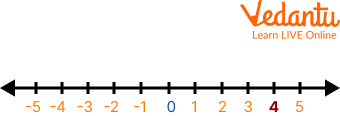Number 4 on the Number Line

## Number 4 with Objects

Number 4 is the counting number. In the below-given images, number 4 is shown by using the objects. Graphics make it easier for the parents and teachers to teach the number 4 to the children.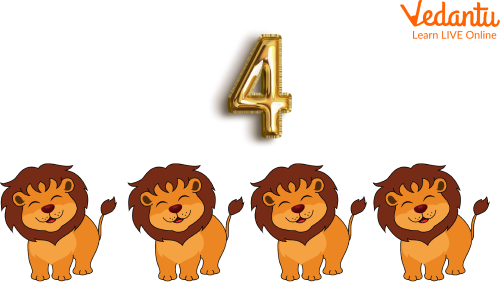Number 4 with Objects

## Count and Write the Number 4

To learn the number 4 easily, one should practice it repeatedly by counting and writing. Some worksheets are given underneath, based on the count and write phenomenon, that will help the children learn the number 4.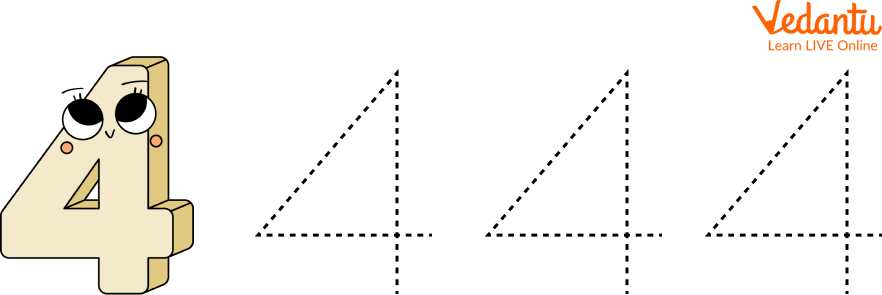Worksheet to Practice Number 4

## Circle the Number 4

Now, the children would have gained a clear picture of the number 4 in their minds which would help them to identify it. The below-given graphics help the kids understand how they need to identify and circle the number 4.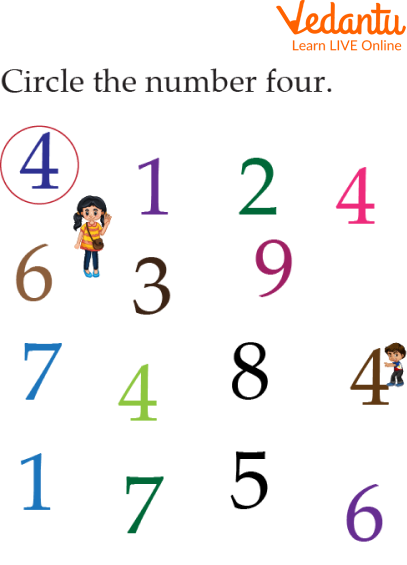Circle the Number 4

## Solved Examples

Q 1. The number four lies between which two numbers?

Ans. As the number 4 is a counting number, it must lie between two numbers. Further, it is greater than the number 3 and less than the number 5. So, it lies between 3 and 5, where 3 is the predecessor of 4 while 5 is its successor.

Thus, the number 4 lies between 3 and 5.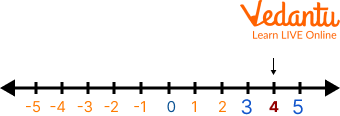Number 4

Q 2. What happens when the number 4 is decreased by 1?

Ans. When the natural number 1 gets subtracted from the number 4, then 4 gets decreased by 1 to its preceding number, 3.

Thus, the number obtained after decreasing from 1, the number 4 is 3.

## Practice Problems

Furthermore, some more worksheets are given, which the children need to complete by themselves. These will help evaluate whether the children can identify the number 4.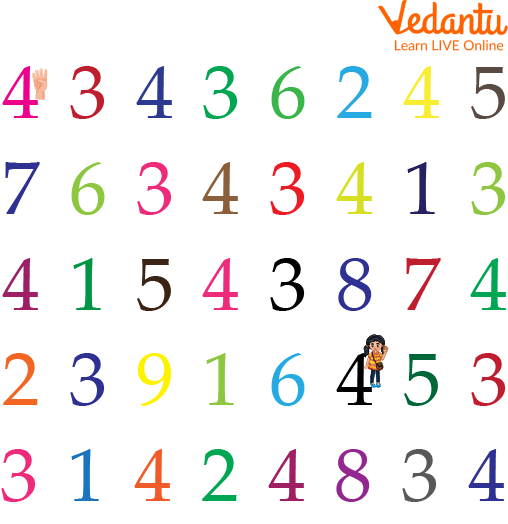Circle the Number 4

## Summary

Summing up here with the topic of learning the number 4. It is the basic building block of memorizing mathematics. It is useful not only in mathematics but also in real life. This article covers every topic related to the number 4 using several graphics so that children can easily grasp the topic. The language used in the writing is kept simple and fascinating to make understanding the concepts easier. I hope you find the article useful and enjoy reading it.

Last updated date: 29th Sep 2023
Total views: 82.2k
Views today: 2.82k

## FAQs on Learn Number 4

1. What is a natural number?

Natural numbers are also called counting numbers. These always start from 1 but do not have any ending point. The English letter, N is used to represent natural numbers.

N = 1, 2, 3, 4, 5, …………

2. What is the number line?

A number line is defined as the visual representation of numbers on a straight or horizontal line. Both ends of the number line point to infinity. One can represent natural numbers, whole numbers, integers, and rational and irrational numbers on the number line. Simply, it can be said that the number line is used to represent real numbers. Sometimes, a number line is also called the 'real line' or 'real number line'.

3. Is 0 included in the set of natural numbers?

No, zero is not included in the set of natural numbers. If the natural numbers are written along with zero, they will form a new set of numbers, called whole numbers, which are represented by the English letter W.